Next: Instabilities Up: Physics of the Ionosphere Previous: Magneto-ionic Theory and the

# Refraction of an HF Radio Wave in the Ionosphere

The SuperDARN radar covers frequencies between 8 and 18 MHz corresponding to wavelengths from 38 to 17 m. These wavelengths are short enough that the ionospheric medium does not change much in the distance of a few wavelengths and can therefore be approximated by a plane stratified medium as indicated in Figure 2.4.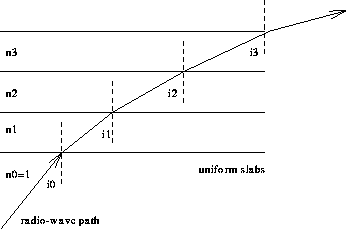Figure 2.4: Refraction of a radio wave in a plane stratified medium

The ionosphere is considered as a stack of thin slabs with refractive indices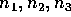etc. Let a plane wave be incident from free space (with refractive index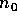) at an angle of incidence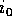. Snell's law can then be applied to each boundary, giving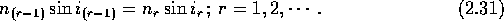Since the plasma frequency increases with height, n becomes smaller (see Equation 2.24) and the ray gradually bends toward the horizontal. This above method of treating radio propagation in a slowly varying ionosphere is the basis of raytracing programs which account for any given electron density profile, the geomagnetic field and collisions. Ray tracing can be done practically with a computer program that solves a set of differential equations.Figure 2.5 shows the results from such a computer program.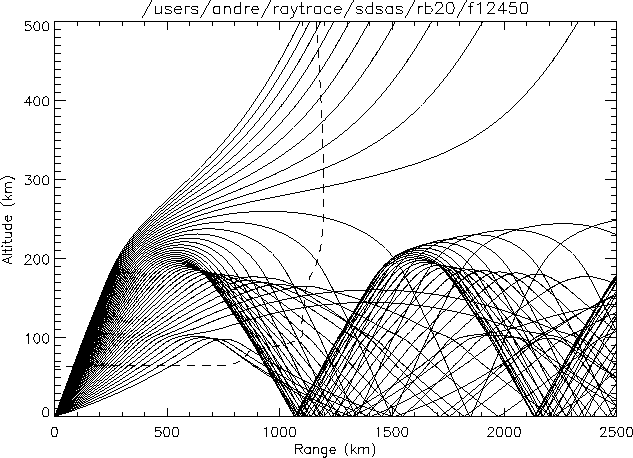Figure 2.5: Side-view of propagation paths of SuperDARN rays, ionosphere at a frequency of 12.45 MHz for elevation angles from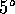to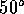. The dashed line indicates the electron density profile. (Dr. D. André, private communication)

Some important features can be identified in Figure 2.5. Rays with large elevation angles propagate through the ionosphere and never return. Rays with small elevation angle can get reflected either at E-layer heights or F-layer heights; these waves will then hit the ground and may be scattered back along the same path to the receiver. These waves are then called groundscatter. Alternatively, these rays can be reflected from the earth back up to the ionosphere and scattering from the ionospheric structures can then occur. The path lengths for these ``hop'' modes can vary from the nominal straight-line propagation from transmitter to scatterer. Since it is generally assumed that the measured range (time delay) can be converted to target position on the basis of straight-line propagation, the distance to the target is generally overestimated. However, extensive calculations have shown that this error is normally no more than one range ``cell'' (45 km) in distance.Next: Instabilities Up: Physics of the Ionosphere Previous: Magneto-ionic Theory and the

Andreas Schiffler
Wed Oct 9 10:05:17 CST 1996